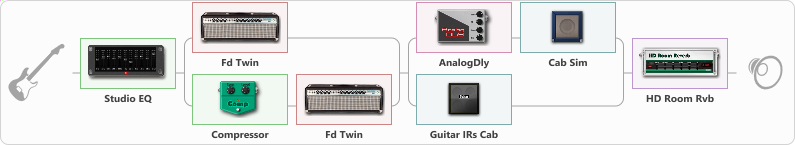# Live Twins

Discussion in 'ToneLib-GFX presets' started by Jean C.Guion, Sep 22, 2020.

1. Live Twins

Preset name: Live Twins

demo'd bias fx2...then made a preset that sounded and felt better...Gfx wins...

Effects chain:Effect: "Studio EQ" (Dynamics / Filter), active - "yes"
{
"31 Hz" = -2
"62 Hz" = -2
"125 Hz" = -3
"250 Hz" = -2
"500 Hz" = 0
"1 kHz" = 2
"2 kHz" = 0
"4 kHz" = 1
"8 kHz" = -3
"16 kHz" = 1
"Level (dB)" = -3
}

Effect: "Splitter" (Dynamics / Filter), active - "yes"
{
"A-Bypass" = Off
"A-Pan" = -100
"A-Level" = 55
"B-Bypass" = Off
"B-Pan" = 100
"B-Level" = 55

'A' branch:
{

Effect: "Fd Twin" (Amp simulators), active - "yes"
{
"Gain" = 67
"Bass" = 50
"Middle" = 50
"Treble" = 50
"Presence" = 51
"Master" = 50
"Level (dB)" = 3
}
}
'B' branch:
{

Effect: "Compressor" (Dynamics / Filter), active - "yes"
{
"Sense" = 37
"Level" = 36
}

Effect: "Fd Twin" (Amp simulators), active - "yes"
{
"Gain" = 33
"Bass" = 46
"Middle" = 51
"Treble" = 43
"Presence" = 54
"Master" = 100
"Level (dB)" = 2
}
}
}

Effect: "Splitter" (Dynamics / Filter), active - "yes"
{
"A-Bypass" = Off
"A-Pan" = -100
"A-Level" = 55
"B-Bypass" = Off
"B-Pan" = 94
"B-Level" = 55

'A' branch:
{

Effect: "AnalogDly" (Delay), active - "yes"
{
"Time" = 1
"Feedback" = 48
"Tone" = 100
"Mix" = 82
}

Effect: "Cab Sim" (Cabinets), active - "yes"
{
"Model" = 2x12" Silver Bell
"Level (dB)" = 0
}
}
'B' branch:
{

Effect: "Guitar IRs Cab" (Cabinets), active - "yes"
{
"Model" = Vox AC30 (2x12")
"Mic Position" = Middle
"Mic Distance" = Middle
"Low Cut (Hz)" = 91
"Hi Cut (kHz)" = 19.5
"Mix" = 100
"Level (dB)" = 0
}
}
}

Effect: "HD Room Rvb" (Reverberation), active - "yes"
{
"Time" = 6.6
"PreLPF" = 83
"PreDelay" = 0
"HiDamp" = 74
"LoGain" = -2.3
"Mix" = 41
}

Note: You will need to download and install the ToneLib-GFX software to use the preset.

File size:
1.1 KB
Views:
2,536